# Finding Graphs of Functions in Nature

Go find functions in nature, and photograph them!

Studying Graphs of Functions?

Well, some graphs of functions appear a lot, so knowing their shapes gives you a much better shot at graphing them correctly and catching common errors.

## What do you need?

• Either a graphing calculator, or a printout of the graphs of functions
• A park or nature trail with plenty of trees or backyard
• A camera
• A keen eye for natural (not human made) shapes

Here are a few examples:f(x) = mx + bf(x) = 1/xf(x) = x2f(x) = √xf(x) = |x|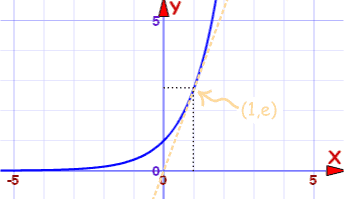f(x) = ex

And here are some example photos we took to give you an idea:

## Graph of f(x) = mx + b

The graph of f(x) = mx+b is a straight line, thus you are looking for something in nature that resembles a straight line. For example: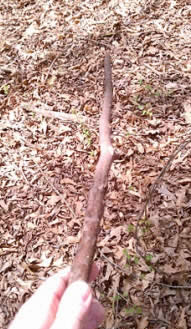TAKE A PIC!

## Graph of f(x) = x2 or -x2

The graph of f(x) = x2 looks like a U, and -x2 is like a ∩, thus you are looking for something that resembles a U or ∩. For example: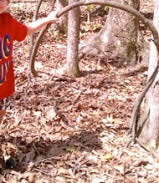TAKE A PIC!

## Graph of Square Root: f(x) = √x

The graph of f(x) = √x looks something like the lower case letter r, for example: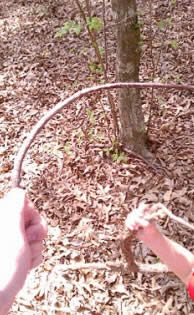TAKE A PIC!

## Graph of Absolute Value: f(x) = |x|

The graph of f(x) = |x| looks some thing like the letter V, for example: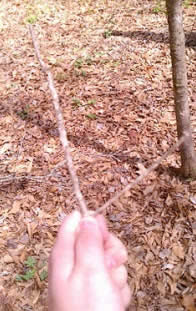TAKE A PIC!

See what other graphs you can find...

When done, compare your pics of nature to the graphs of functions and put together a presentation.

Activity courtesy of Hands On Math